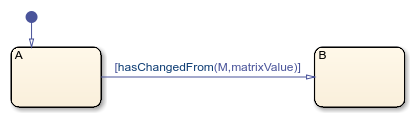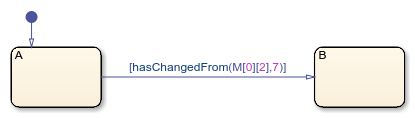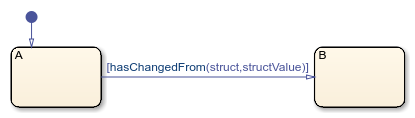# hasChangedFrom

Detect change in data from specified value

## Syntax

``tf = hasChangedFrom(data_name,value)``

## Description

example

````tf = hasChangedFrom(data_name,value)` returns 1 (`true`) if the value of `data_name` was equal to the specified `value` at the beginning of the previous time step and is a different value at the beginning of the current time step. Otherwise, the operator returns 0 (`false`).The argument `data_name` can be: A scalar variable.A matrix or an element of a matrix.If `data_name` is a matrix, the operator returns `true` when it detects a change in any element of `data_name` and the previous value of `data_name` was equal to `value`.Index elements of a matrix by using numbers or expressions that evaluate to a constant integer. See Supported Operations for Vectors and Matrices.A structure or a field in a structure.If `data_name` is a structure, the change detection operator returns `true` when it detects a change in any element of `data_name` and the previous value of `data_name` was equal to `value`.Index fields in a structure by using dot notation. See Index and Assign Values to Stateflow Structures.Any valid combination of structure fields or matrix elements. The argument `data_name` cannot be a nontrivial expression or a custom code variable. NoteStandalone charts in MATLAB® do not support change detection on an element of a matrix or a field in a structure. The argument `value` can be any expression that resolves to a value that is comparable with `data_name`: If `data_name` is a scalar, then `value` must resolve to a scalar value.If `data_name` is a matrix, then `value` must resolve to a matrix value with the same dimensions as `data_name`.Alternatively, in a chart that uses C as the action language, `value` can resolve to a scalar value. The chart uses scalar expansion to compare `data_name` to a matrix whose elements are all equal to the value specified by `value`. See Assign Values to All Elements of a Matrix.If `data_name` is a structure, then `value` must resolve to a structure value whose field specification matches `data_name` exactly. ```

## Examples

expand all

Transition out of state if the previous value of the matrix `M` was equal to `matrixValue` and any element of `M` has changed value since the last time step or input event.

`[hasChangedFrom(M,matrixValue)]`Transition out of state if the element in row 1 and column 3 of the matrix `M` has changed from the value `7` since the last time step or input event.

In charts that use MATLAB as the action language, use:

`[hasChangedFrom(M(1,3),7)]`In charts that use C as the action language, use:

`[hasChangedFrom(M,7)]`Transition out of state if the previous value of the structure `struct` was equal to `structValue` and any field of `struct` has changed value since the last time step or input event.

`[hasChangedFrom(struct,structValue)]`Transition out of state if the field `struct.field` has changed from the value `5` since the last time step or input event.

`[hasChangedFrom(struct.field,5)]`## Tips

• If multiple input events occur in the same time step, the `hasChangedFrom` operator can detect changes in data value between input events.

• If the chart writes to the data object but does not change the data value, the `hasChangedFrom` operator returns `false`.

• The type of Stateflow® chart determines the scope of the data supported by the change detection operators:

• Standalone Stateflow charts in MATLAB: `Local` only

• In Simulink® models, charts that use MATLAB as the action language: `Input` only

• In Simulink models, charts that use C as the action language: `Input`, `Output`, `Local`, or ```Data Store Memory```

• In a standalone chart in MATLAB, a change detection operator can detect changes in data specified in a call to the `step` function because these changes occur before the start of the current time step. For example, if `x` is equal to zero, the expression `hasChangedFrom(x,0)` returns `true` when you execute the chart `ch` with the command:

`step(ch,'x',1);`
In contrast, a change detection operator cannot detect changes in data caused by assignments in state or transition actions in the same time step. Instead, the operator detects the change in value at the start of the next time step.

• In a chart in a Simulink model, if you enable the chart option Initialize Outputs Every Time Chart Wakes Up, using an output as the argument of the `hasChanged` operator always returns `false`. For more information, see Initialize outputs every time chart wakes up.

• When row-major array layout is enabled in charts that use `hasChangedFrom`, code generation produces an error. Before generating code in charts that use `hasChangedFrom`, enable column-major array layout. See Select Array Layout for Matrices in Generated Code.